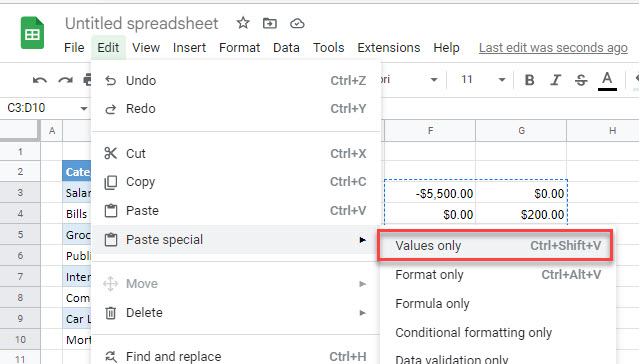# Change / Reverse Signs of Values in Excel & Google Sheets

This tutorial demonstrates how to change the signs of values in Excel and Google Sheets.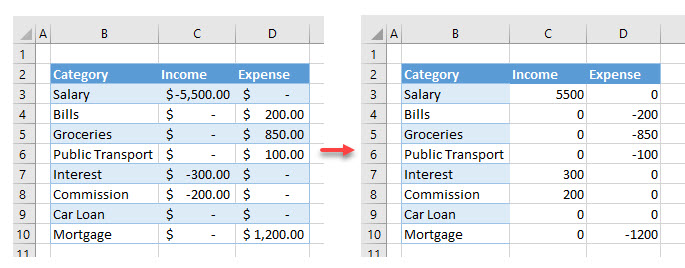## Reverse Value Signs

If you want to change (reverse) the signs of values in Excel (from negative to positive, or from plus to minus), you can achieve it easily using Paste Special. Say you have the following data with Income in Column C and Expense in Column D.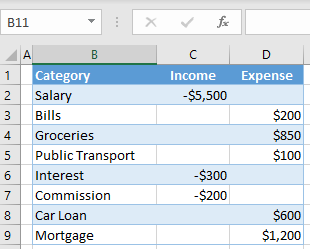As shown, income values have negative signs while expenses are positive. To correct this and change signs, follow these steps:

1. Multiply by a constant with Paste Special. First enter -1 in an empty cell (here, cell F2).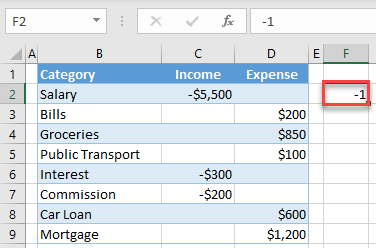1. Right-click the cell with the value -1 (cell F2), and select Copy (or use the keyboard shortcut CTRL + C).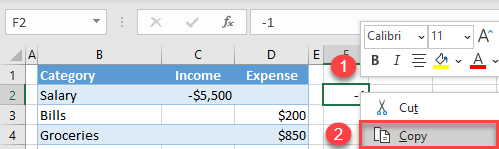1. Select and right-click the range where you want to reverse signs (here, C2:D9), and choose Paste Special.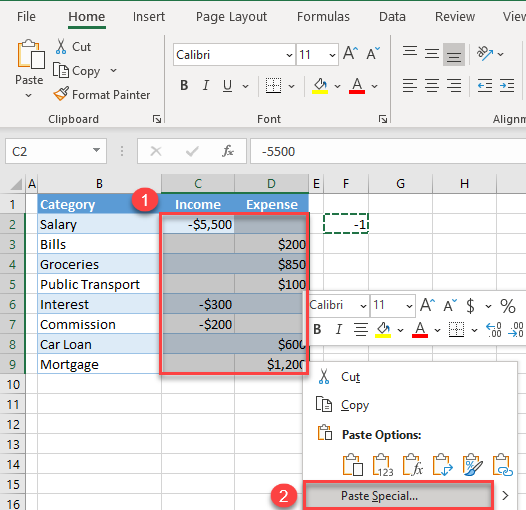1. In the Paste Special window, select Multiply, and click OK.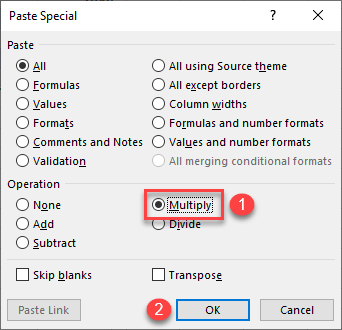As a result, all values from the paste range are multiplied by the copied value (-1), and signs are reversed.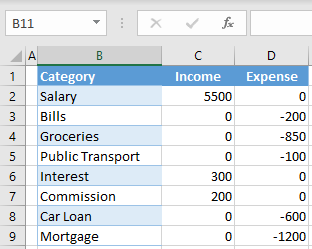Since multiply is not a Paste Special option in Google Sheets, you need to use formulas to reverse the signs in Google Sheets.

For example, to reverse the amount in C2 you can (in a blank cell) use the formula

`` =-C2``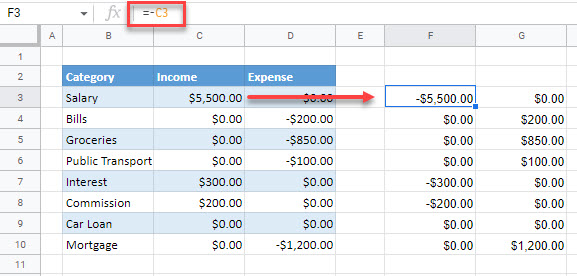You would then need to copy this formula down for the required rows, and across to the next column to reverse all the values.

To convert the formulas to values, you can copy the formulas and then use Paste special > Values only.